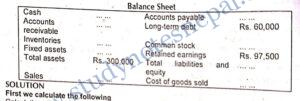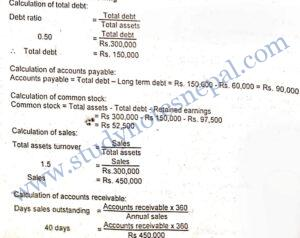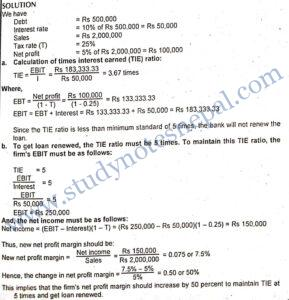###### 1. Who are some of the basic users of financial statements and how do they use them?

Financial statements contain a balance sheet, income statement, cash flow statement and the statement of retained earnings of a firm. Various stakeholders use the firm’s financial statement. The basic users of financial statements are shareholders, creditors, bankers, suppliers, managers, employees and government. These stakeholders are interested in monitoring the firm and to ensure that their interests are being served. They rely on the company’s financial statements for necessary information. They use financial statements to analyze the financial strengths and weaknesses associated with various aspects of a firm’s performance for their own purposes. For example, as an insider, the management shows greater concern about the overall financial strength and weakness of the firm. Similarly, shareholders analyze the financial statements to have information about the earnings of the company. Short-term creditors analyze financial statements to know about short-term solvency positions of the firm. They are more eager to know the current debt payment capacity of a firm. Similarly, other institutional lenders and bondholders are concerned with fixed charge payment capacity of the firm. Thus, the purpose of using financial statements varies according to specific interests of the different stakeholders.

###### 2.  What is free cash flow? Why is it required for managerial decision making?

Free cash flow is the cash flow remaining after satisfying investments in fixed assets, new products, and working capital necessary to sustain ongoing operations. It is defined as the net operating profit after (NOPAT) tax minus investment in working capital and fixed assets necessary to sustain the business. It is calculated as:

FCF = NOPAT – Net investment in operating capital

The net cash flows cannot be maintained over time because a firm has to replace its depreciating fixed assets and also has to develop new products. Hence, firms are not completely free to use the net cash flows. The firm is required to use some of its cash flow to satisfy the investment in operating capital. Thus, free cash flow is the amount of cash flow left after meeting the investment need of new operating capital. In financial decision making, the financial manager should relay on free cash flow to determine the value of the firm. In other words, the value of the firm depends on all the future expected free cash flows (FCF). Higher the future expected free cash flow higher will be the value of the firm. Thus free cash flow is required for every managerial decision.

However, it should be noted that a firm might have positive net operating profit after tax but a negative free cash flow. It may be due to high investments in operating assets needed to support growth. In such cases the firm is supposed to rely on external financing to satisfy the part of investment not covered by net operating profile after taxes. Such a situation is not bad. On the other hand, FCF may be negative because of negative NOPAT. If this is so, the firm is probably experiencing operating problems and hence a need for improvement in the firm’s operation is necessary.

###### 3. What difference do you find between asset management ratios and debt management ratios?

Assets management ratios are used to assess productivity of a firm’s assets in generating sales revenue whereas debt management ratios are used to assess the firm’s efficiency in utilizing debt capital. The fundamental differences between these two types of ratios are as follows:

 Assets Management Ratios Debt Management Ratios Assets management ratios are used to evaluate the productive power of firm’s investment in different assets Debt management ratios are used to assess the use of debt capital by firms. Assets management ratios show whether the firm has held adequate investment in productive assets. Debt management ratios show the extent of use of debt capital to finance the total assets Assets management ratios show the productivity of assets. Debt management ratios show the long-term solvency position of the firm. Assets management ratios show the qualitative liquidity position of a firm’s investment in inventories and receivables. Debt management ratios show the firm’s debt serving and fixed charge coverage capacity. Assets management ratios show the efficiency of asset structure invested by firms. Debt management shows the efficiency of capital structure employed by the firm.
###### 4. What are the limitations of ratio analysis? Explain.

Financial ratios are powerful tools in analyzing the firm’s financial statement. However, they should be used with extreme care; they suffer from some limitations. Some specific limitations of ratio analysis are as follows:

• It requires a basis of comparison: All financial ratios require a base for comparison to derive meaningful conclusions about a firm’s financial performance. For example, a firm may have a return on assets of 10 percent. It has to be compared with the ratio of similar firms or the ratio of industry averages to conclude on the profitability performance of the firm.
• It suffers from differences in interpretation: Ratio analysis does not only involve the calculation of financial ratios but also the meaningful interpretation of the calculated ratios. However, different analysts may interpret the same ratios in different ways. For example, the use of preferred stock either as debt or as equity is determined by the purpose of analysis. Long-term debt holders include preferred stock as a part of equity while stockholders may treat preferred stock as a part of debt.
• Ratios are not comparable if two firms operate under different situations: When we compare the ratio of one firm with the ratio of other firms, the comparison may not be justifiable. The reason is that the situation under which one firm is being operated may differ from that of another firm. Similarly, the situation of a firm itself may be different at different points of time.
• Suffers from change in price level: Financial ratio analysis uses accounting figures in financial statements. The accounting figures are stated in monetary values, which are assumed to remain constant. But in practice, prices do not remain constant; they go on changing as per price level changes.
• It actually does not indicate the future: Financial analysts use financial ratios to form a judgment of a firm’s financial position and performance in future. However, as they are calculated on the basis of historical accounting information, they simply suggest what happened in the past rather than what is going to happen in future.

In using different ratios, qualitative considerations are also necessary along with the quantitative indication. For example, the calculated current ratio of a firm is 2.5 times and quantitatively it is preferable from a liquidity point of view. However, if a larger part of the accounts receivable comprising a great portion of current assets may be uncollectible and of no value. Thus the qualitative aspect also deserves consideration.

###### 5. Why is the Du-Pont system of analysis useful in analyzing a firm’s performance? Explain.

Du-Pont system is a comprehensive method of financial analysis first used and popularized by Du-Pont Corporation. It is used to make classified assessments of the link between a firm’s profitability, operating efficiency, assets management and debt management. A simple Du-Pont system links return on a firm’s assets as a function of operating efficiency measured by net profit margin and the assets productivity measured by total assets turn over. It is stated as:

ROA=Profit Margin x Assets Turnover

By using this simple Du-Pont equation, ratio analysts can assess how a firm’s return on assets is affected by profit margin and assets turnover. Profit margin and assets turnover have a multiplier effect on a firm’s return on assets. For example, given a profit margin of a firm, if assets turnover increases the return on assets also increases proportionally. Putting in other words, for a given level of assets turnover, the return on assets increases proportionally with profit margin. Thus by using a simple Du-Pont system of financial analysis, the analyst can understand the contributing factor for a given level of return on asset.

Another Du-Pont equation is in expanded form which links firm’s return on equity with profit margin, assets turnover and equity multiplier. It is expressed as follows: ROE Profit margin x Assets turnover Equity multiplier

According to the extended DU-Pont equation, a firm’s return on equity depends on operating efficiency denoted by profit margin, assets utilization denoted by assets turnover and use of debt and equity indicated by equity multiplier. Besides profit margin and assets turnover, a firm’s return on equity is also affected by equity multiplier. The equity multiplier represents the use of debt by a firm. Other things held constant, use of higher debt than equity increases equity multiplier and thus also increases return on equity. But if a firm with higher equity multiplier has lower operating efficiency its profit margin will also be lower causing ROE to decline. If the firm’s investments in assets are not productively utilized to generate sales revenue, the assets turnover also will be lower resulting in lower ROE. Thus by using an extended form of the Du-Pont system of financial analysis, financial analysts can make classified assessments of the causing factors for a given level of ROE.

###### 6. What are the uses of financial ratios to stakeholders? Explain.

Various stakeholders of a firm use financial ratios to analyze the financial statements for their own interest and purpose. The basic users of financial ratios are shareholders, managers, creditors, banks and financial institutions, competitors and so on. The uses of financial ratios to these stakeholders can be stated as follows:

• As insiders, management is more concerned about the overall financial strength and weaknesses of the firm. Therefore, management uses financial ratios to assess liquidity, assets management, debt management and profitability of the firm for the purpose o. financial decision making.
• Shareholders analyze the financial statements to have information about the earnings of the firm. They are interested to know how earnings per share, dividend per share, rate of return on equity has been growing over the years. They are also interested to know the value created by the company over the years so they use several financial ratios for these purposes.
• Not only shareholders but also the short and long-term creditors, institutional lenders, bondholders and government are interested in analyzing financial statements. Short-term creditors carry out financial ratio analysis to know about short-term solvency positions of the firm. They are more eager to know the current debt payment capacity of a firm. Similarly, other institutional lenders and bondholders are concerned with fixed charge payment capacity of the firm.

Thus, the type of financial ratio analysis undertaken varies according to specific interests of the users.

## Numerical Problems

1. Nepal Trading Company had earnings per share of Rs.4 last year, and it paid a Rs.2 dividend. Book value per share at year-end was Rs.40, while total retained earnings increased by Rs.12 million during the year. The company has no preferred stock, and no new common stock was issued during the year. If the company’s year-end debt (which equals its total liabilities) was Rs.120 million, what was the company’s year-end debt/assets ratio?

SOLUTION

Given,

Earning per share (EPS). = Rs 4

Dividend per share (DPS) = Rs 2

Book value per share (BVPS) = Rs 40

Increase in retained earnings = Rs 12 million

Total debt =  Rs 120 million

We know,

Retained earnings per share EPS-DPS = Rs 4 – Rs 2 = Rs 2

Number of shares outstanding = Total increase in retained earnings /Retained earnings per share

=Rs 12 million/ Rs 2

= 6 million

Equity Number of outstanding shares x BVPS= 6 million x Rs 40 = Rs 24

Total assets Total debt Equity = Rs 120 + Rs 240 = Rs 360

Now, we calculate debt-asset ratio as follows:

Debt-asset ratio = Total debt/ Total assets

= Rs 120 million /Rs 360 million = 0.3333 or 33.33%

2. Lalitpur Brick Company has Rs. 1,313,500 in current assets and Rs. 525,000 in current liabilities. Its initial inventory level is Rs. 375,000 and it will raise funds as additional notes payable and use them to increase inventory. How much can a company’s short term debt (notes payable) increase without violating a current ratio of 2 to 1? What will be the firm’s quick ratio after the company has raised the maximum amount of short-term funds?

SOLUTION

We have,

Current assets= Rs 1,312,500

Current liabilities= Rs 525,000

Initial inventory level = Rs 375,000

Let us suppose the firm raises Rs x in notes payable to finance the additional inventories of equal amount. With this, the increase in inventories is equal to the increase in current liabilities, and the firm’s current ratio after the increase can be defined as follows:

Current ratio = Rs 1,312,500 + Rs x / Rs 525,000 + Rs x

2= Rs 1,312,500 + x / Rs 525,000+ Rs x

Rs 1,050,000+ 2x =Rs 1,312,500 + Rs x

2x-x= Rs 1,312,500 – Rs 1,050,000

x = Rs 262,500

Hence, the firm should raise Rs 262,500 in notes payable to increase inventories of the equal amount to maintain the current ratio at 2 times.

Now, with this increase in notes payable and increase in inventories, the firm will have:

New total current assets = Rs 1,312,500 + Rs 262,500 = Rs 1,575,000

New total current liabilities = Rs 525,000 + Rs 262,500 = Rs 787,500

New quick ratio = = Rs 1,575,000- (Rs 375,000 + Rs 262,500) / Rs 787,500

= 1.19 times

Thus the quick ratio will be 1.19 times after the firm has raised the maximum amount of short term funds in notes payable.

3. From the following data of a certain company, calculate current assets.

 Cash and marketable securities   Fixed assets   Sales   Net income   Quick ratio   Current ratio   DSO   ROE Rs. 100 million   283.50 million   1,000 million   50 million   2.0 times   3.0 times   40 days   12%

SOLUTION

Calculation of accounts receivable:

We know,

DSO  = Accounts receivable x 360 days / Sales

40 = Accounts receivable x 360 days / Rs 1,000

Accounts receivable = 40 x Rs 1000 / 360

= Rs 111.11 million.

Calculation of current liabilities:

We know,

Quick ratio =

2.0 =  Rs 100+ Rs 111.11/ Current liabilities

Current liabilities = (Rs 100+ Rs 111.11)/2 = Rs 105.56 million.

Calculation of current assets:

We know,

Current Ratio =

3.0  =Current assets/ Rs 105.56

current assets: = 3 x Rs 105.56 = Rs 316.68 million.

4. Dhaulagiri Match Company had a quick ratio of 1.4, a current ratio of 3.0, an inventory turnover of 6 times, total current assets of Rs 810,000, and cash and marketable securities of Rs 120,000 in 1998. What were the company’s annual sales and its Days Sales Outstanding (DSO) for that year?

SOLUTION

Given,

Quick ratio= 1.4

Current ratio= 3.0

Inventory turnover= Rs 810,000

Total current assets= 6 times

Cash and marketable securities = Rs 120,000

Calculation of current liabilities:

Current ratio =

3.0 =

Current liabilities =

= Rs.270,000

Calculation of inventory:

Quick ratio =

1.4 =

Rs. 387,000 = Rs. 810,000 – Inventory

Inventory = Rs. 432,000,

Calculation of accounts receivable:

Receivable = Current assets – Cash & marketable securities – Inventory

= Rs. 810,000 – Rs.120,000 – Rs. 432,000 = Rs. 258,000.

Now, we calculate sales as follows:

Inventory turnover = Sales / Inventory

6 = Sales / Rs.432,000

Sales = Rs. 2,592,000

And, we calculate DSO as follows:

Days sales outstanding (DSO) = (Receivable )/Annual Sales/360

=Rs.258,000 /Rs.2,592,000/360

=Rs. 258,000 / Rs.7,200

= Rs.270,000

5. Complete the balance sheet and sales information in the table that follows for Iceberg Industries using the following financial data:

Debt ratio  50%

Quick ratio    0.8x

Total assets turnover 1.5x

Days sales outstanding 40 days

Gross profit margin on sales30%

Inventory turnover 5xSOLUTION6. (Old)Garment Corporation has Rs 500,000 of debt outstanding, and it pays an interest rate of 10 percent annually. Corporations’ annual sales are Rs 2,000,000, its average tax rate is 25 percent; and its net profit margin on sales is 5 percent. If the corporation does not maintain a TIE ratio of at least 5 times, its bank will refuse to renew the loan, and bankruptcy will result.

1. What is the corporation’s TIE ratio? b. By what percentage net profit margin should increase in order to get a loan renewed?